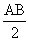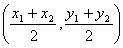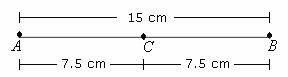Definition Of Midpoint

Midpoint of a line segment is the point that is halfway between the endpoints of the line segment.

A line segment has only one midpoint.
If AB is a line segment and P is the midpoint, then AP = BP =.
In two-dimensional coordinate plane, the midpoint of a line with coordinates of its endpoints as (x1, y1) and (x2, y2) is given byExamples Of MidpointIn the above figure, length ofis 15 cm and distance of C from both the endpoints A andB is 7.5 cm. So, C is the midpoint ofA. (1, - 4)
B. (- 1, 4)
C. (1, 4)
D. (- 1, - 4)